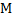# The value ofat a particular point is. Suppose the earth suddenly shrinks uniformly to half its present size without losing any mass. The value ofat the same point (assuming that the distance of the point from the centre of earth does not shrink) will now be a)b)c)d)## Question ID - 55318 :- The value ofat a particular point is. Suppose the earth suddenly shrinks uniformly to half its present size without losing any mass. The value ofat the same point (assuming that the distance of the point from the centre of earth does not shrink) will now be a)b)c)d)3537

 (c). Sinceandare constant, soThe potential energy of 4-particalse each of mass 1 kg placed at the four vertices of a square of side length 1 m is a)b)c)d)